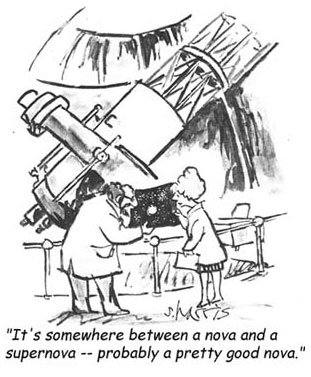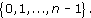Approximations are most useful when it comes to making rough estimates –
Author(s): The Open University

Sometimes it doesn’t make sense to round to a specific number of decimal places. If, say, you were calculating the cost of fencing at £10.65 per metre, for a garden boundary, the length of which had been given to you as 185 feet, then you would want to multiply 10.65 × 185 × 0.3048. (Conversion of feet to metres was given in Author(s): The Open University

• 1.   The condition ‘is equal to’ is a relation on any set of real numbers because, for any x, y in the set, the statement ‘x is equal to y’ is either definitely true or definitely false. This relation is usually denoted by the symbol =. For this relation, each real number in the set is related only to itself!

• 2.   The condition ‘is less than’ is a relation on any set of real numbers, and we usually
Author(s): The Open University

The Division Algorithm tells us that all the possible remainders on division by an integer n lie in the setWe denote this set by [Image_Link]https://www.open.edu/
Author(s):
The Open University

We will now discuss complex numbers and their properties. We will show how they can be represented as points in the plane and state the Fundamental Theorem of Algebra: that any polynomial equation with complex coefficients has a solution which is a complex number. We will also define the function exp of a complex variable.

Earlier we mentioned several sets of numbers, including [Image_Link]https://www.open.edu/openlearn/ocw/pluginfile.php/89651/mod_ouc
Author(s):
The Open University## Study another free course

There are more than 800 courses on OpenLearn for you to
Author(s): The Open University

An important idea when calculating volumes of simple shapes is that of a cross-section. In the case of the rectangular box considered above, it is possible to slice through the box horizontally so that the sliced area is exactly the same as the area of the base or top; in other words, the areas of the horizontal cross-sections are equal.

[Image_Link]https://www.open.edu/openlearn/ocw/pluginfile.php/94675/mod_oucontent/ouco
Author(s): The Open University

What is a volume? The word usually refers to the amount of three-dimensional space that an object occupies. It is commonly measured in cubic centimetres (cm3) or cubic metres (m3).

A closely related idea is capacity; this is used to specify the volume of liquid or gas that a container can actually hold. You might refer to the volume of a brick and the capacity of a jug – but not vice versa. Note that a container with a particular volume will not nec
Author(s): The Open University

## Question 1

Find the area of a circle of (a) radius 8 cm, and (b) radius 15 m.

• (a)

Author(s): The Open University

## Question 1

Find the area of each of these shapes.

[Image_Link]https://www.open.edu/openlearn/ocw/pluginfile.php/94675/mod_oucontent/oucontent/779/39f826a0
Author(s): The Open University

## Question 1

Calculate all the angles at the centres of these objects.

Here is an improved solution which shows working.

## Example 13

Suppose you plan to redecorate your bathroom. The end wall has the following shape, with dimensions as shown on the diagram. The quality of the plasterwork is not good and you are considering tiling the wall.

Author(s): The Open University

In this type of question you are given the answer! All the marks are allocated for correct reasoning and justification.

## Example 15

Suppose you now decide to place your new bath (length 1.7 m, height 0.8 m) against this wall as shown in the diagram below.

Author(s): The Open University

Consider some of the many different things we can do with language: express ourselves in metaphor, issue commands, ask questions, fill in crosswords, write shopping lists and diary entries, repeat nursery rhymes by rote, solve logical or arithmetical problems, make promises, tell stories, sign our names, etc. Impressive though it is, this variety in the uses of language is a potential distraction from our main interest, which is in the use of language to represent. It will therefore help if w
Author(s): The Open University

Section 6 contains solutions to the exercises that appear throughout sections 1-5.

Click the link below to open the solutions (13 pages, 232KB).

Section 6

Author(s): The Open University

Fractions and decimals can also be converted to percentages, by multiplying by 100%.

So, for example, 0.17, 0.3 andcan be expressed as percentages as follows:

0.17 × 100% = 17%;

<
Author(s): The Open University

Ratios crop up often in official statistics. The government wants the teacher–pupil ratio in schools to be increased to one teacher to thirty pupils or less. The birth rate has fallen: the ratio of children to women of child bearing age has gone down. It used to be 2.4 to 1, and now it is 1.9 to 1. Predictions for the ratio of working adults to retired adults is disturbing. Predictions are, that by 2030 the ratio will be two working adults to every retired person, instead of three to one no
Author(s): The Open University

The content acknowledged below is Proprietary (see and conditions made available under a Creative Commons Attribution-NonCommercial-ShareAlike 4.0 Licence) and used under licence.

Course image: rod
Author(s): The Open University

Mathematical processes are different from content in that they overarch the subject and are not thought of as hierarchical. A list of processes could contain:

• problem-solving (including investigating);

• mathematical modelling;

• reasoning;

• communicating;

• making connections (including applying mathematics); and

• using tools.

Each of the six processes listed here repre
Author(s): The Open University